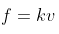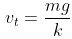×
Log in to StudySoup
Get Full Access to University Physics - 13 Edition - Chapter 5 - Problem 106p
Join StudySoup for FREE
Get Full Access to University Physics - 13 Edition - Chapter 5 - Problem 106p

Already have an account? Login here
×
Reset your password

# CALC You throw a rock downward into water with a speed ofISBN: 9780321675460 31

## Solution for problem 106P Chapter 5

University Physics | 13th Edition

• Textbook Solutions
• 2901 Step-by-step solutions solved by professors and subject experts
• Get 24/7 help from StudySoup virtual teaching assistantsUniversity Physics | 13th Edition

4 5 1 379 Reviews
31
0
Problem 106P

CALC? You throw a rock downward into water with a speed of ?3mg/k?, where k is the coefficient in Eq. (5.5). Assume that the relationship between fluid resistance and speed is as given in Eq. (5.5), and calculate the speed of the rock as a function of time.

Step-by-Step Solution:

Problem 106P

CALC? You throw a rock downward into water with a speed of 3mg/k?, where k is the coefficient in Eq. (5.5). Assume that the relationship between fluid resistance and speed is as given in Eq. (5.5), and calculate the speed of the rock as a function of time.

Step By Step Solution

Step 1 of 3

The resistance force affecting the rock is given by:The rock reaches maximum velocity when the force of resistance due to water becomes equal to its weight. That velocity os known as terminal velocity.....................(1)

Step 2 of 3

Step 3 of 3

##### ISBN: 9780321675460

Unlock Textbook Solution

Enter your email below to unlock your verified solution to:

CALC You throw a rock downward into water with a speed of